# C語言中的文件操作實例分析

### 2.什么是文件

#### 2.1程序文件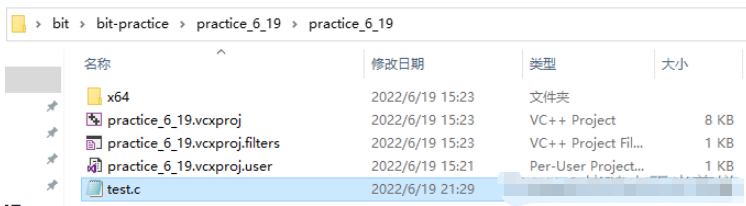### 3.文件的打開和關閉

#### 3.1文件指針

```struct?_iobuf?{
????char?*_ptr;
????int??_cnt;
????char?*_base;
????int??_flag;
????int??_file;
????int??_charbuf;
????int??_bufsiz;
????char?*_tmpfname;
???};
typedef?struct?_iobuf?FILE;```

FILE* pf://文件指針變量

?定義pf是一個指向FILE類型數據的文件指針變量，可以使pf指向某個文件的文件信息區（一個結構體變量），通過該文件信息區中的信息就能夠訪問該文件，也就是說，通過文件指針變量能夠找到與他關聯的文件。#### 3.2文件的打開和關閉

ANSI C規定使用fopen函數來打開文件，使用fclose函數來關閉文件。

FILE* fopen(const char* filename, const char* mode);

int fclose (FILE* stream);?```#include?<stdio.h>
int?main()
{
//打開文件
FILE*?pf?=?fopen("test.txt",?"w");
if?(pf?==?NULL)//遇到錯誤時函數會返回NULL指針
{
perror("fopen");
return?1;
}
//文件操作
//?
//關閉文件
fclose(pf);
pf?=?NULL;//把文件指針置空，防止野指針的問題
return?0;
}```

```int?main()
{
//打開文件
FILE*?pf?=?fopen("test.txt",?"r");
//文件操作
//?
//關閉文件
fclose(pf);
pf?=?NULL;//把文件指針置空，防止野指針的問題
return?0;
}```### 4.文件的順序讀寫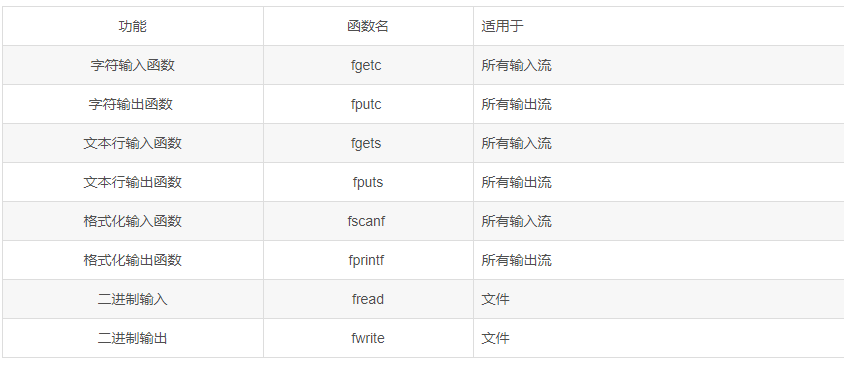• 標準輸入流：stdin，指的是鍵盤

• 標準輸出流：stdout，指的是屏幕

• 標準錯誤流：stderr，也是屏幕

int? fputc(int c, FILE* stream);

fputc會返回被輸出字符的ASCII碼值，遇到錯誤時返回EOF。?

```#include?<stdio.h>
int?main()
{
//打開文件
FILE*?pf?=?fopen("test.txt",?"w");
if?(pf?==?NULL)
{
perror("fopen");
return?1;
}
//寫文件
char?ch?=?0;
for?(ch?=?'a';?ch?<=?'z';?ch++)
{
fputc(ch,?pf);
}
//關閉文件
fclose(pf);
pf?=?NULL;
return?0;
}```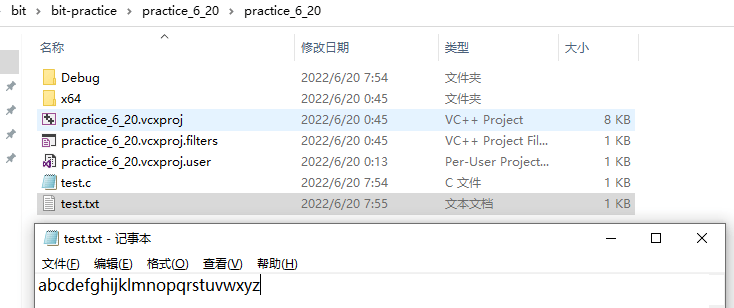int fgetc(FILE* stream);

fgetc會返回讀到的字符的ASCII碼值，當讀到文件末尾或者遇到錯誤時會返回EOF。

```int?main()
{
//打開文件
FILE*?pf?=?fopen("test.txt",?"r");
if?(pf?==?EOF)
{
perror("fopen");
return?1;
}
//讀文件?-?輸入操作
int?ch?=?0;
while?((ch?=?fgetc(pf))?!=?EOF)
{
printf("%c?",?ch);
}
//關閉文件
fclose(pf);
pf?=?NULL;
return?0;
}``````int?main()
{
int?ch?=?fgetc(stdin);
fputc(ch,?stdout);
return?0;
}```

int fputs(const char* string, FILE* stream);

```int?main()
{
//打開文件
FILE*?pf?=?fopen("test1.txt",?"w");
if?(pf?==?NULL)
{
perror("fopen");
return?1;
}
//寫文件
fputs("abcdefg\n",?pf);
fputs("hijklmn\n",?pf);
//關閉文件
fclose(pf);
pf?=?NULL;
return?0;
}```

?此時打開test1.txt查看數據：?char* fgets(char* string, int n, FILE* stream);

```int?main()
{
//打開文件
FILE*?pf?=?fopen("test1.txt",?"r");
if?(pf?==?NULL)
{
perror("fopen");
return?1;
}
//寫文件
char?arr?=?{?0?};
while?(fgets(arr,?256,?pf)?!=?NULL)
{
fputs(arr,?stdout);
}
//關閉文件
fclose(pf);
pf?=?NULL;
return?0;
}```

int fprintf( FILE *stream, const char *format [, argument ]...);?

```struct?S
{
char?name;
int?age;
double?score;
};
?
int??main()
{
struct?S?s?=?{?"zhangsan",?20,?75.5?};
//打開文件
FILE*?pf?=?fopen("test2.txt",?"w");
if?(pf?==?NULL)
{
perror("fopen");
return?1;
}
//寫文件
fprintf(pf,?"%s?%d?%f",?s.name,?s.age,?s.score);
//關閉文件
fclose(pf);
pf?=?NULL;
return?0;
}```?同樣的，stream位置的參數可以是標準輸出流，這樣數據就會輸出到屏幕上面來了

```struct?S
{
char?name;
int?age;
double?score;
};
?
int??main()
{
struct?S?s?=?{?"zhangsan",?20,?75.5?};
fprintf(stdout,?"%s?%d?%f",?s.name,?s.age,?s.score);
return?0;
}```

int fscanf( FILE *stream, const char *format [, argument ]... );?

fscanf和scanf和很像，只是scanf默認stream位置的參數是標準輸入流，也就是鍵盤。

```int??main()
{
struct?S?s?=?{0};
//打開文件
FILE*?pf?=?fopen("test2.txt",?"r");
if?(pf?==?NULL)
{
perror("fopen");
return?1;
}
//讀文件
fscanf(pf,?"%s?%d%?lf",?s.name,?&(s.age),?&(s.score));
fprintf(stdout,?"%s?%d?%lf",?s.name,?s.age,?s.score);
//關閉文件
fclose(pf);
pf?=?NULL;
return?0;
}```

?將數據從文件上讀取，然后在屏幕上輸出。

?再來認識兩個與printf和scanf很像的函數：

sprintf：將格式化的數據轉換為字符串

int sprintf( char *buffer, const char *format [, argument] ... );

?sscanf：將字符串轉換為格式化的數據

int sscanf( const char *buffer, const char *format [, argument ] ... );

sprintf的使用：

```struct?S
{
char?name;
int?age;
double?score;
};
?
int?main()
{
char?buf?=?{?0?};
struct?S?s?=?{?"wangwu",?30,?85.5?};
sprintf(buf,?"%s?%d?%lf",?s.name,?s.age,?s.score);
printf("%s\n",?buf);
return?0;
}```?sscanf的使用：

```struct?S
{
char?name;
int?age;
double?score;
};
?
int?main()
{
char?buf?=?{?0?};
struct?S?s?=?{?"wangwu",?30,?85.5?};
sprintf(buf,?"%s?%d?%lf",?s.name,?s.age,?s.score);
//將字符串轉化為格式化的數據
struct?S?tmp?=?{?0?};
sscanf(buf,?"%s?%d?%lf",?tmp.name,?&(tmp.age),?&(tmp.score));
printf("%s?%d?%lf",?tmp.name,?tmp.age,?tmp.score);
return?0;
}```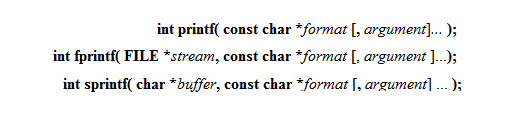?二進制輸出函數：

size_t fwrite( const void *buffer, size_t size, size_t count, FILE *stream );

• buffer：指向被讀取的數據的指針

• size：被讀取的項目的字節大小

• count：被讀取的項目數的最大值

• stream：文件流

fwrite的使用：

```struct?S
{
char?name;
int?age;
double?score;
};
?
int?main()
{
struct?S?s?=?{?"lisi",?15,?95.5?};
//打開文件
FILE*?pf?=?fopen("test3.txt",?"wb");
if?(pf?==?NULL)
{
perror("fopen");
return?1;
}
//寫文件
fwrite(&s,?sizeof(struct?S),?1,?pf);
//關閉文件
fclose(pf);
pf?=?NULL;
return?0;
}```

?因為是以二進制的方式寫的，所以文件中存放的數據是這樣子的：size_t fread( void *buffer, size_t size, size_t count, FILE *stream );?

```struct?S
{
char?name;
int?age;
double?score;
};
?
int?main()
{
struct?S?s?=?{?0?};
//打開文件
FILE*?pf?=?fopen("test3.txt",?"rb");
if?(pf?==?NULL)
{
perror("fopen");
return?1;
}
//讀文件
printf("%s?%d?%lf\n",?s.name,?s.age,?s.score);
//關閉文件
fclose(pf);
pf?=?NULL;
return?0;
}```

### 5.文件的隨機讀寫

#### 5.1fseek

int fseek( FILE *stream, long offset, int origin );

• stream：文件流

• offset：相對于此時文件指針的位置的偏移量，單位是字節

• origin：文件指針此時的位置

origin可以分為3個值：

SEEK_CUR - 文件指針當前的位置

SEEK_SET - 文件開始的位置

SEEK_END - 文件末尾的位置

```#include?<stdio.h>
int?main()
{
FILE*?pf?=?fopen("test.txt",?"w");
if?(pf?==?NULL)
{
perror("fopen");
return?1;
}
//把a到f的字符寫入文件
char?ch?=?0;
for?(ch?=?'a';?ch?<=?'f';?ch++)
{
fputc(ch,?pf);
}
fclose(pf);
pf?=?NULL;
return?0;
}```

fseek的使用：

```int?main()
{
FILE*?pf?=?fopen("test.txt",?"r");
if?(pf?==?NULL)
{
perror("fopen");
return?1;
}
//讓文件指針從此時的位置向后移動5個字節
fseek(pf,?5,?SEEK_CUR);
int?ch?=?fgetc(pf);
printf("%c\n",?ch);
fclose(pf);
pf?=?NULL;
return?0;
}```offset輸入負值也可以讓指針往前走

```int?main()
{
FILE*?pf?=?fopen("test.txt",?"r");
if?(pf?==?NULL)
{
perror("fopen");
return?1;
}
//讓文件指針從文件尾的位置向前移動3個字節
fseek(pf,?-3,?SEEK_END);
int?ch?=?fgetc(pf);
printf("%c\n",?ch);
fclose(pf);
pf?=?NULL;
return?0;
}```#### 5.2ftell

long int ftell ( FILE * stream );

ftell的使用：?

```int?main()
{
FILE*?pf?=?fopen("test.txt",?"r");
if?(pf?==?NULL)
{
perror("fopen");
return?1;
}
fseek(pf,?0,?SEEK_END);
long?size??=?ftell(pf);
printf("%ld\n",?size);
fclose(pf);
pf?=?NULL;
return?0;
}```

#### 5.3rewind

void rewind( FILE *stream );?

rewind的使用：

```int?main()
{
FILE*?pf?=?fopen("test.txt",?"r");
if?(pf?==?NULL)
{
perror("fopen");
return?1;
}
fseek(pf,?0,?SEEK_END);
//從文件尾回到文件起始位置
rewind(pf);
long?size??=?ftell(pf);
printf("%ld\n",?size);
fclose(pf);
pf?=?NULL;
return?0;
}```

### 6.文本文件和二進制文件

?根據數據的儲存形式，數據文件被稱為文本文件和二進制文件。```int?main()
{
FILE*?pf?=?fopen("test.txt",?"w");
if?(pf?==?NULL)
{
perror("fopen");
return?1;
}
int?a?=?10000;
fwrite(&a,?sizeof(int),?1,?pf);//二進制的形式寫
fclose(pf);
pf?=?NULL;
return?0;
}```?由于該編譯器是小端字節序存儲，所以可以看到16進制顯示的數字是反過來的。

### 7.文件讀取結束的判定

#### 7.1被錯誤使用的feof

fgetc函數判斷返回值是否為EOF

fgets函數判斷返回值是否為NULL

feof和ferror函數

feof

int feof( FILE *stream );?

?文件是由于讀取到文件尾結束時返回一個非0值，反之返回0

ferror

int ferror( FILE *stream );

```int?main()
{
int?c;?//?注意：int，非char，要求處理EOF
FILE*?fp?=?fopen("test.txt",?"r");
if?(fp?==?NULL)
{
perror("fopen");
return?1;
}
//fgetc?當讀取失敗的時候或者遇到文件結束的時候，都會返回EOF
while?((c?=?fgetc(fp))?!=?EOF)?//?標準C?I/O讀取文件循環
{
putchar(c);
}
//判斷是什么原因結束的
if?(ferror(fp))
else?if?(feof(fp))
puts("End?of?file?reached?successfully");
fclose(fp);
fp?=?NULL;
return?0;
}```

```enum
{?
SIZE?=?5?
};
?
int?main(void)
{
double?a[SIZE]?=?{?1.,2.,3.,4.,5.?};
FILE*?fp?=?fopen("test.txt",?"wb");
if?(fp?==?NULL)
{
perror("fopen");
return?1;
}
fwrite(a,?sizeof?*?a,?SIZE,?fp);?//?寫?double?的數組
fclose(fp);
fp?=?NULL;
?
double?b[SIZE];
fp?=?fopen("test.txt",?"rb");
if?(ret_code?==?SIZE)?
{
for?(int?n?=?0;?n?<?SIZE;?++n)?
printf("%f?",?b[n]);
putchar('\n');
}
if?(feof(fp))//判斷是否因為遇到文件尾造成的文件讀取結束
else?if?(ferror(fp))?//判斷是否因為讀取失敗造成的文件讀取結束
{
}
fclose(fp);
fp?=?NULL;
}```

### 8.文件緩沖區

ANSIC標準采用“緩沖文件系統”處理的數據文件的，所謂緩沖文件系統是指系統自動地在內存中為程序中每一個正在使用的文件開辟一塊“文件緩沖區”。從內存向磁盤輸出數據會先送到內存中的緩沖區，裝滿緩沖區后才一起送到磁盤上。如果從磁盤向計算機讀入數據，則從磁盤文件中讀取數據輸入到內存緩沖區（充滿緩沖區），然后再從緩沖區逐個地將數據送到程序數據區（程序變量等）。緩沖區的大小根據C編譯系統決定的。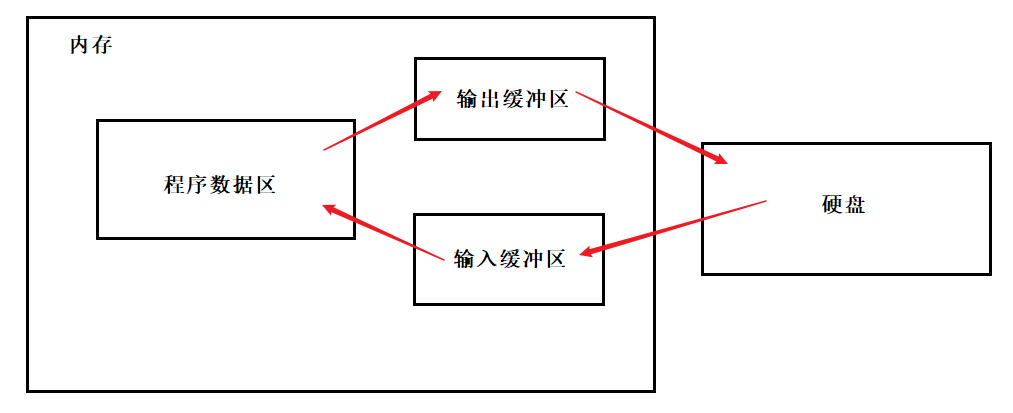```int?main()
{
FILE*?pf?=?fopen("test.txt",?"w");
if?(pf?==?NULL)
{
perror("fopen");
return?1;
}
fputs("abcdef",?pf);//先將代碼放在輸出緩沖區
printf("睡眠10秒-已經寫數據了，打開test.txt文件，發現文件沒有內容\n");
Sleep(10000);
printf("刷新緩沖區\n");
fflush(pf);//刷新緩沖區時，才將輸出緩沖區的數據寫到文件（磁盤）
printf("再睡眠10秒-此時，再次打開test.txt文件，文件有內容了\n");
Sleep(10000);//此時睡眠10秒，是為了說明數據是由于fflush的刷新才輸出到文件中的
fclose(pf);
//注：fclose在關閉文件的時候，也會刷新緩沖區
pf?=?NULL;
return?0;
}```

fflush函數可以讓數據不充滿緩沖區時就直接輸入或者輸出

#### 評論

2018人人澡人摸人人添_月夜影视在线观看资源_一本二卡三卡四卡乱码小说_tobu8在线观看下载